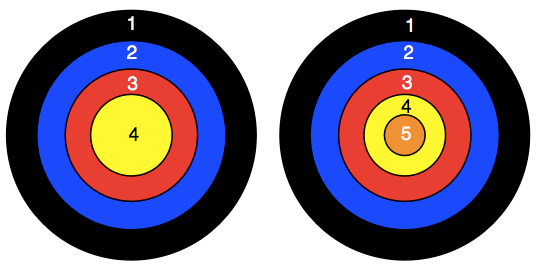#### You may also like### Prompt Cards

These two group activities use mathematical reasoning - one is numerical, one geometric.### Consecutive Numbers

An investigation involving adding and subtracting sets of consecutive numbers. Lots to find out, lots to explore.### Exploring Wild & Wonderful Number Patterns

EWWNP means Exploring Wild and Wonderful Number Patterns Created by Yourself! Investigate what happens if we create number patterns using some simple rules.

# Dart Target

##### Age 7 to 11Challenge Level
We have two different targets and a set of three darts.You use three darts for each game.  All the darts always hit the target.

### CHALLENGE 1

Each time you have a game, you add up the score of the three darts.
Your challenge is to have four games each with the same total score, but made in different ways.

However, note that 1 and 2 on the left target and 3 on the right target is the same as 1 on the left target and 2 and 3 on the right target, so is counted as just one solution.

### CHALLENGE 2

Have three games. The three totals must give three consecutive numbers.
In the nine darts used no number must occur more than twice.
So, when Raj chooses:

Game 1:     2 + 3 + 4 =  9
Game 2:     1 + 4 + 5 = 10
Game 3:     1 + 5 + 5 = 11

This is NOT allowed as three 5s have been used and the maximum is two.

### CHALLENGE 3

You now move on to having three targets with four darts for each game.
As before you can only have one dart in any coloured sector of a target.

The targets are:
1, 2, 3
1, 2, 3, 4
1, 2, 3, 4, 5.

Firstly see how many different totals you can make.

Finally find all the ways of getting the set of three consecutive 11, 12, 13

But in a set of three answers you must not use the same number more than three times.

So, when Sara chooses:

Game 1   1 + 2 + 3 + 5 =  11
Game 2   1 + 2 + 4 + 5  = 12
Game 3   2 + 2 + 4 + 5 =  13

This is not allowed as four 2s have been used and the maximum is three.

Find as many answers as you can.